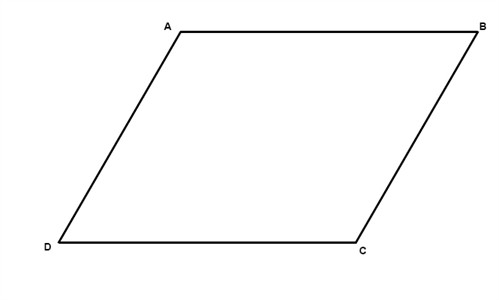# Check whether four points make a parallelogram

• Difficulty Level : Basic
• Last Updated : 22 Jun, 2022

Given four points in a 2-dimensional space we need to find out whether they make a parallelogram or not.

A parallelogram has four sides. Two opposite sides are parallel and are of same lengths.Examples:

```Points = [(0, 0),  (4, 0),  (1, 3),  (5, 3)]
Above points make a parallelogram.

Points = [(0, 0), (2, 0), (4, 0), (2, 2)]
Above points does not make a parallelogram
as first three points itself are linear.```

Problems for checking square and rectangle can be read from Square checking and Rectangle checking but in this problem, we need to check for the parallelogram. The main properties of the parallelogram are that opposite sides of parallelogram are parallel and of equal length and diagonals of parallelogram bisect each other. We use the second property to solve this problem. As there are four points, we can get total 6 midpoints by considering each pair. Now for four points to make a parallelogram, 2 of the midpoints should be equal and rest of them should be different. In below code, we have created a map, which stores pairs corresponding to each midpoint. After calculating all midpoints, we have iterated over the map and check the occurrence of each midpoint, If exactly one midpoint occurred twice and other have occurred once, then given four points make a parallelogram otherwise not.

## CPP

 `// C++ code to test whether four points make a``// parallelogram or not``#include ``using` `namespace` `std;` `// structure to represent a point``struct` `point {``    ``double` `x, y;``    ``point() { }``    ``point(``double` `x, ``double` `y)``        ``: x(x), y(y) { }` `    ``// defining operator < to compare two points``    ``bool` `operator<(``const` `point& other) ``const``    ``{``        ``if` `(x < other.x) {``            ``return` `true``;``        ``} ``else` `if` `(x == other.x) {``            ``if` `(y < other.y) {``                ``return` `true``;``            ``}``        ``}``        ``return` `false``;``    ``}``};` `// Utility method to return mid point of two points``point getMidPoint(point points[], ``int` `i, ``int` `j)``{``    ``return` `point((points[i].x + points[j].x) / 2.0,``                ``(points[i].y + points[j].y) / 2.0);``}` `// method returns true if point of points array form``// a parallelogram``bool` `isParallelogram(point points[])``{``    ``map > midPointMap;` `    ``// looping over all pairs of point to store their``    ``// mid points``    ``int` `P = 4;``    ``for` `(``int` `i = 0; i < P; i++) {``        ``for` `(``int` `j = i + 1; j < P; j++) {``            ``point temp = getMidPoint(points, i, j);` `            ``// storing point pair, corresponding to``            ``// the mid point``            ``midPointMap[temp].push_back(point(i, j));``        ``}``    ``}` `    ``int` `two = 0, one = 0;` `    ``// looping over (midpoint, (corresponding pairs))``    ``// map to check the occurrence of each midpoint``    ``for` `(``auto` `x : midPointMap) {``        ` `        ``// updating midpoint count which occurs twice``        ``if` `(x.second.size() == 2)``            ``two++;``        ` `        ``// updating midpoing count which occurs once``        ``else` `if` `(x.second.size() == 1)``            ``one++;``        ` `        ``// if midpoint count is more than 2, then``        ``// parallelogram is not possible``        ``else``            ``return` `false``;    ``    ``}` `    ``// for parallelogram, one mid point should come``    ``// twice and other mid points should come once``    ``if` `(two == 1 && one == 4)``        ``return` `true``;``    ` `    ``return` `false``;``}` `// Driver code to test above methods``int` `main()``{``    ``point points;` `    ``points = point(0, 0);``    ``points = point(4, 0);``    ``points = point(1, 3);``    ``points = point(5, 3);` `    ``if` `(isParallelogram(points))``        ``cout << ``"Given points form a parallelogram"``;``    ``else``        ``cout << ``"Given points does not form a "``                ``"parallelogram"``;``    ``return` `0;``}`

Output:

`Given points form a parallelogram`

Time Complexity: O(p2logp) , where p is number of points
Auxiliary Space: O(p2), where p is number of points